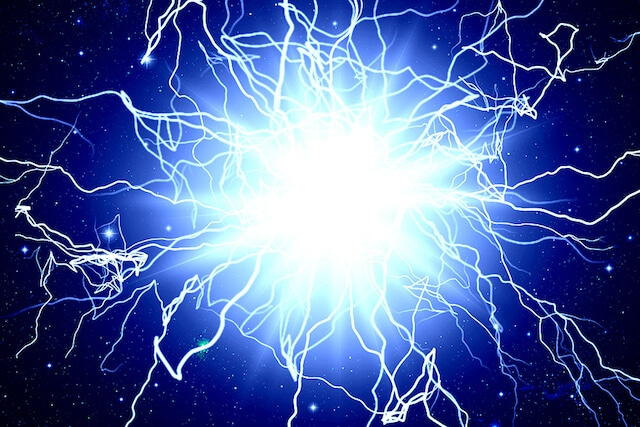Energy

The energy of a system is defined as its ability to do work. The “work” here refers to the precise definition of “work done” in physics, which is the product of the amount of force applied on an object, and the displacement of that object. Basically, if it can apply force and cause movement, it has energy.

Energy is a scalar quantity (SI Unit: J) that comes in many forms. A single system may contain many forms of energy at once. For example, your phone is powered by electrical energy, possesses gravitational potential energy, and releases thermal and light energy. That’s a lot of types of energy!

In general, energy can be classified as potential and kinetic energy. Kinetic energy is the energy possessed by a body by virtue of its movement, while potential energy is the energy possessed by a body due to its position or state. Here are some of the types of energy and what they mean:

 Type of energy Description Kinetic energy Energy of an object as a result of its motion. Light energy A form of electromagnetic radiation that is visible to the human eye. It is released in the form of photons, usually when an electron drops from a higher energy state to a lower one. Thermal energy Energy possessed by a body due to the vibration of its atoms and molecules. It is related to temperature. Electrical energy Energy resulting from the flow of charged particles, i.e. electrons. Nuclear energy Energy stored in the nucleus of atoms. It is released during a nuclear fission or fusion reaction, usually done in nuclear power plants to generate electricity. Chemical potential energy Energy stored in the chemical bonds between atoms, within molecules. Gravitational potential energy Energy stored in an object due to its position in a gravitational field, such as the gravitational attraction of the Earth. Elastic potential energy Energy stored in an elastic object that has been stretched or compressed.

Potential and kinetic energy

A moving object possesses both potential and kinetic energy. As such, it is imperative that you know how to derive both quantities. Kinetic energy can be calculated from the mass and velocity of the object that is in motion.

• Formula: Kinetic energy = ½ x Mass (kg) x (Velocity (m/s))2
• Simplified formula: KE = ½ mv2
• SI Unit: Joule (J)

The potential energy which you will deal with the most in physics is gravitational potential energy. This can be derived using the mass of the object, the gravitational field strength, and height above a reference point (usually the vertical height from the ground).

• Formula: Gravitational potential energy = Mass (kg) x Gravitational field strength (N/kg) x Height (m)
• Simplified formula: GPE = mgh
• SI Unit: Joule (J)

(On Earth, the gravitational field strength is about 10 N/kg)

Energy conversion and conservation

Just as important as the types of energy is the conversion of energy. One of the fundamental concepts in the study of energy conversion and conservation is the Principle of Conservation of Energy. This principle states that the total amount of energy in an enclosed system remains constant. By extension, energy can neither be created nor destroyed. It can only be transferred from one body to another, or converted to another form.

In practice, this means that when dealing with any action or process, the total energy at the start should be identical to the total energy at the end. In the context of mechanical energy, which is the sum of kinetic and potential energy, this means that in ideal conditions:

• Total Initial Mechanical Energy = Total Final Mechanical Energy
• KE1 + GPE1 = KE2 + GPE2
• KE1 = Initial Kinetic Energy
• GPE1 = Initial Gravitational Potential Energy
• KE2 = Final Kinetic Energy
• GPE2 = Final Gravitational Potential Energy

However, most processes do not occur in isolation. Mechanical energy is often lost to the environment after being converted to other forms of energy, like light, heat, or sound energy. As such, in practice, the equation looks more like this:

• KE1 + GPE1 = KE2 + GPE2 + energy converted

Machines are also subject to this loss of energy. When dealing with machines, the energy that is converted to undesirable types of energy is referred to as ‘wasted’ energy output. Since you usually want to maximise the amount of ‘useful’ energy output, it is important to know how to calculate the efficiency of a machine.

• Efficiency = (Useful Energy Output / Total Energy Input) x 100%
• Efficiency = (Useful Power Output / Total Power Input) x 100%

Conclusion

In this article, we have discussed the types of potential and kinetic energy, as well as the fundamental concepts of energy conversion and conservation. These concepts are inextricably linked to other concepts in classical mechanics, like work and power.

If you need help understanding how these topics link and work in tandem, a competent physics tuition centre can lend a hand. Here at Best Physics Tuition, we can guide you through your physics journey, and push you to excel in your O Level and A Level physics.